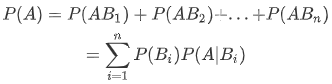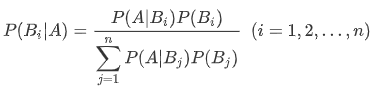## 1.条件概率与乘法公式

• 条件概率
事件$B$已发生的条件下，事件$A$发生的概率。记为$P(A|B)$
条件概率$P(A|B)$反映了事件的先后主从关系。• 条件概率公式
\begin{equation}
P(A|B)=\frac{P(AB)}{P(B)}
\end{equation}
• 乘法公式
\begin{equation}
P(AB)=P(A|B)P(B)=P(B|A)P(A)
\end{equation}
• 乘法公式推广
\begin{equation}
P(A_1A_2A_3)=P((A_1A_2)A_3)=P(A_1A_2)P(A_3|(A_1A_2))=P(A_1)P(A_2|A_1)P(A_3|A_1A_2)
\end{equation}

## 2.全概率公式（知因求果）

• 划分
事件$B_1,B_2,...,B_n$为样本空间$\Omega$的自制件，且满足以下两个条件:则称$B_1,B_2,...,B_n$为样本空间$\Omega$的以组划分。• 全概率公式
$P(B_i)>0,(i=1,2,3,...,n)$，且$A\subset\Omega$，则有:• 注意
1.所求事件的概率受到多个因素的影响。
2.分析清楚哪些事件对A有概率贡献，所以一定要注意把温杯时间组描述清楚。
3.全概率公式中要用到的条件概率，一般是根据实际情况直接得到的。

## 3.贝叶斯公式（执果探因）## 4.事件的独立性

• 两个事件独立
• 定义1
对于事件A、B，若有$P(A)P(B)=P(AB)$，则称事件A与B相互独立，简称独立。
• 性质
若事件A与B独立，那么$A$$\overline{B}$$B$$\overline{A}$$\overline{A}$$\overline{B}$也独立。
• 定理1
如果四对事件$A$,$B$$A$,$\overline{B}$$\overline{A}$,$B$$\overline{A}$,$\overline{B}$之中有一对相互独立，则另外三队也相互独立。
• 三个事件独立
• 定义2
如果事件A、B、C满足下列条件:
1.$P(ABC)=P(A)P(B)P(C)$
2.$P(AB)=P(A)P(B)$
$\space \space P(AC)=P(A)P(C)$
$\space \space P(BC)=P(B)P(C)$
则称A、B、C是相互独立的。如果仅有2式成立，则称事件A、B、C是两两独立的。
相互独立一定两两独立，反之不然
• n个事件独立
• 定义3
设有$n$个事件$A_1,A_2,A_3,...,A_n$，若对其中任意$m(2\le m\le n)$个事件$A_{i1},A_{i2},...,A_{im}$都有$P(A_{i1}A_{i2}...A_{im})=P(A_{i1})P(A_{i2})...P(A_{im})$，则称事件$A_1,A_2,...,A_n$相互独立。
• 定理2
$n$个事件$A_1,A_2,...,A_n$相互独立，则其中任意$m(2\le m\le n)$个事件也相互独立，将其中任意$m(1\le m\le n)$个事件换成对立事件后仍相互独立。
• 注意
1.相互独立$\not =$互不相容
2.相互独立则两两独立，反之不一定成立。

## 5.伯努利概型

• 特点
1.试验可重复$n$次。
2.每次试验只有两个可能结果$A,\overline{A}$
3.$n$次试验相互独立——每次试验结果与其它次试验无关。
$n$重伯努利试验中事件$A$出现$k$次的概率记为:
\begin{equation}
P_{\color{red}n}(k)
\end{equation}
• 二项概率公式
一般地，二项概率公式:
\begin{equation}
P(A)=p,\space P(\overline{A})=1-p=q
\end{equation}
$n$重伯努利试验中$A$恰好发生$k$次的概率:
\begin{equation}
P_n(k)=\color{red}{C_n^k}p^kq^{n-k}
\end{equation}
$P_n(k)$可视为二项式$(p+q)^n$展开式中的一般想，故称为二项概率公式。

## 发送评论编辑评论

|´・ω・)ノ
ヾ(≧∇≦*)ゝ
(☆ω☆)
（╯‵□′）╯︵┴─┴
￣﹃￣
(/ω＼)
∠( ᐛ 」∠)＿
(๑•̀ㅁ•́ฅ)
→_→
୧(๑•̀⌄•́๑)૭
٩(ˊᗜˋ*)و
(ノ°ο°)ノ
(´இ皿இ｀)
⌇●﹏●⌇
(ฅ´ω`ฅ)
(╯°A°)╯︵○○○
φ(￣∇￣o)
ヾ(´･ ･｀｡)ノ"
( ง ᵒ̌皿ᵒ̌)ง⁼³₌₃
(ó﹏ò｡)
Σ(っ °Д °;)っ
( ,,´･ω･)ﾉ"(´っω･｀｡)
╮(╯▽╰)╭
o(*////▽////*)q
＞﹏＜
( ๑´•ω•) "(ㆆᴗㆆ)

Emoji